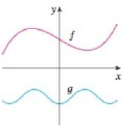Chapter 1.1, Problem 70E### Single Variable Calculus: Early Tr...

8th Edition
James Stewart
ISBN: 9781305270343

#### Solutions

Chapter
Section### Single Variable Calculus: Early Tr...

8th Edition
James Stewart
ISBN: 9781305270343
Textbook Problem

# Graphs of f and g are shown. Decide whether each function is even, odd, or neither. Explain your reasoning.70.To determine

To decide: Whether the graph of the functions f(x) and g(x) is odd, even or neither.

Explanation

Definition used:

A function is said to be an even when its graph is symmetric about the y-axis.

A function is said to be an odd when its graph is symmetric about the origin.

Notice that the graph of f(x) is neither symmetric about the origin nor symmetric about the y-axis.

Therefore, the graph of f(x) is neither even nor odd

### Still sussing out bartleby?

Check out a sample textbook solution.

See a sample solution

#### The Solution to Your Study Problems

Bartleby provides explanations to thousands of textbook problems written by our experts, many with advanced degrees!

Get Started

#### In Exercises 516, evaluate the given quantity. log218

Finite Mathematics and Applied Calculus (MindTap Course List)

#### In Problems 35-42, simplify each complex fraction. 40.

Mathematical Applications for the Management, Life, and Social Sciences

#### n=12(14)n converges to: a) 94 b) 2 c) 23 d) the series diverges

Study Guide for Stewart's Single Variable Calculus: Early Transcendentals, 8th